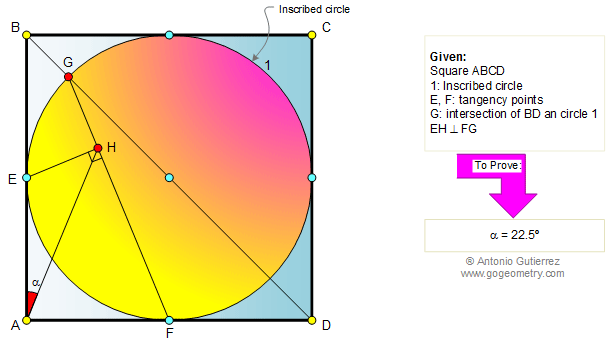# Geometry Problem 373: Square, Inscribed Circle, Diagonal, Perpendicular, Angle

 The figure shows a circle 1 inscribed in a square ABCD of area S. Points E and F are the tangency point. G is the intersection point of BD and circle 1 and EH is perpendicular to FG. Prove that the measure of angle EAH is 22.5 degrees. .Home | Search | Geometry | Problems | All Problems | 371-380 | Semicircle | Perpendicular Chords | Circle Inscribed in a Square | Email | View or post a solution